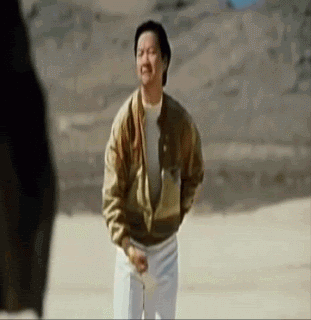ברוך הבא אורח ( התחבר | הירשם )Bible Black 02 encoding scriptzor אפשרויותSirSwagALot22.07.2013, 08:41 הודעה #1חבר ANBUקבוצה: וותיקים הודעות: 3,058 תאריך הצטרפות: 8.05.06 מיקום: בלגיה משתמש מספר: 10,131First - pixor for comparison:Before:After:Encoding script:d2vpath = "VTS_01_1.d2v"MPEG2Source(d2vpath,info=3)#Rainbow-Kill pre-IVTCBifrost(Interlaced=true, scenelumathresh=5) #Very solid, good and tried De-Rainbower, I use it pre-IVTC.#DotCrawl-Kill pre-IVTCTComb(mode=0,fthreshL=3,othreshL=5) #TComb is multifunctional, in this mode I use it to kill some light Dot-Crawl.#Pre-IVTC Denoising + Sharpening and then some denoisingfft3dfilter(sigma=1.4, bt=5, bw=32, bh=32, ow=16, oh=16, sharpen=0.2,interlaced=true,wintype=1, degrid=1)#IVTC(InVerseTele-Cine[Weave 2 fields to 1 frame, and cut out the repeating frames by decimation{29.970--->23.976}])Interp = nnedi(field=-1)deint = TDeint(mode=0,Order=-1,field=-1,type=1,AP=55,edeint=interp,slow=2,emask=TMM(mode=0,order=-1,field=-1))tfm(d2v=d2vpath,clip2=deint,order=-1,Mode=5, pp=7, field=-1, slow=2).TDecimate(mode=1, denoise=true)#Color Correction(used for color conversion from TV colors to PC colors[Well, not precisely "color" conversion, also the levels of brightness/darkness])SmoothLevels(input_low=16, gamma=1, input_high=250, output_low=0, output_high=255,preset="tv2pc", limiter=2,lmode=0,protect=19,chroma=100)#Cropping and Resizing - killing off useless borders and resizing to a resolution which will give correct PC aspect ratio.Crop(10,2,-6,-2) #Crop a bit, but kep AR as close to 1.5 as you can(X:Y=1.5), here we got 1.496, acceptable.BlackManResize(784,576) #Correct resize to preserve Aspect Ratio from TV to PC resolution, on NTSC 4:3 will be X:Y = 1.3625 ---> 784/576=1.361, which is close enought.crop(8, 0, -8, -0) #Then we crop to make it 4:3 PC standard, which is X:Y = 1.3333#Light single-grain Denoising, like the old-days UnDot()RemoveGrain(mode=1)#Semi-Heavy DenoiserVagueDenoiser(threshold=0.8, method=1, nsteps=6, chromaT=2.0)#Line DarkeningToon(strength=0.3)#Line Thinning and small sharpeningWarpSharp(64, 10, 64, -0.6)#Picture SharpeningLSFmod(Strength=70, Smode=3, SMethod=3,Secure=true, Lmode=-1, Soft=-2, Soothe=true, Keep=70).LimitedSharpenFaster(Smode=4, strength=50)#DeHalo - kills that white hallow around the line-edges.DeHalo_alpha_mt(rx=2.0, ry=2.0, darkstr=0.5, brightstr=0.7, lowsens=47, highsens=53, ss=1.5)#AntiAliasing - helps get rid of broken lines and straighten shit out, but also smoothens shit and kills details - careful usage is required.MAA(mask=2)#Heavy Denoiser, smoothenerhqdn3d(1.3,1.3,1.3,1.3)LimitedSharpenFaster(Smode=4, strength=30)Coming out soon. If my translator isn't a lazy ass. --------------------Laught at a struggling writer: Druids and shit1 משתמשים נמצאים בנושא זה (1 אורחים ו-0 משתמשים אנונימים)
0 משתמשים:גרסת ארכיון הזמן כרגע: 22.08.2019 , 00:11